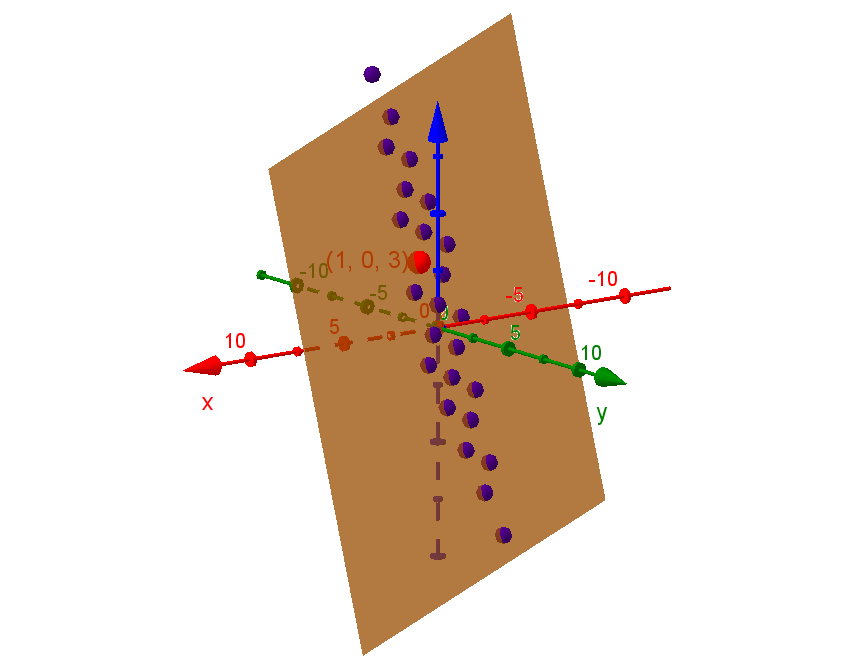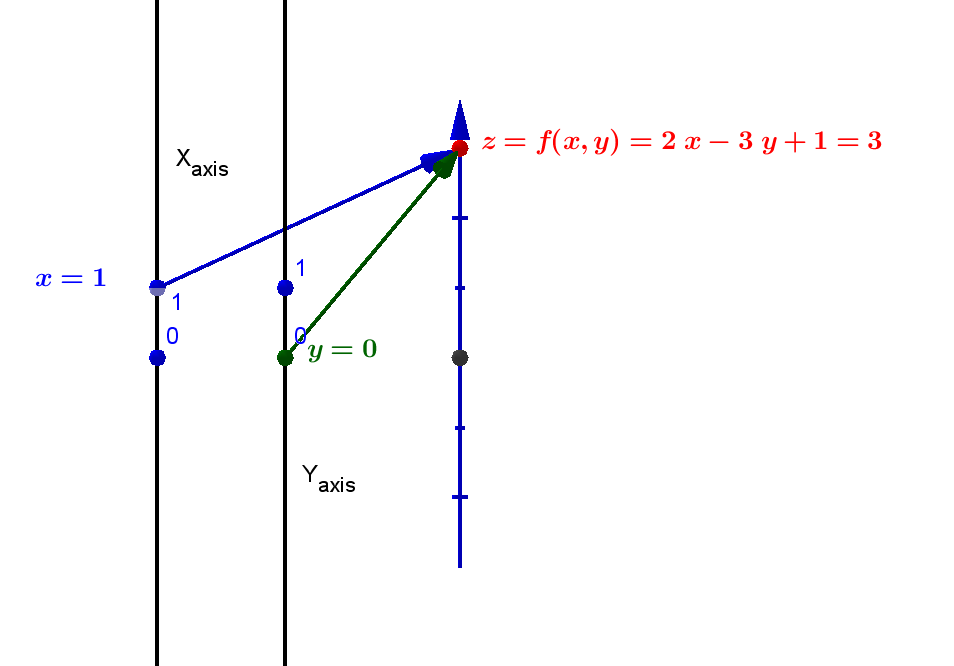##### Example MV.TMD.0:  Suppose \$z\$ is a function of \$x\$ and \$y\$ given by the equation \$z = f (x,y) = 2x - 3y + 1\$.

 \$x\$ \$y\$ -2 -1 0 1 2 2 -9 -7 -5 -3 -1 1 -6 -4 -2 0 2 0 -3 -1 1 3 5 -1 0 2 4 6 8 -2 3 5 7 9 11

\$f(x, y) = 2x - 3y + 1\$
Table
MV.TMD1\$f(x, y) = 2x - 3y + 1\$
3D
Graph
MV.TMD1\$f(x, y) = 2x - 3y + 1\$
Mapping Diagram
MV.TMD1

Table MV.TMD1 shows a table of selected values for this function.
The same function is visualized in 3D Graph MV.TMD1.
Mapping Diagram MV.TMD1 visualizes only the data that \$f(1,0)=3\$.

In Graph TMD1, the graph of the table values of the function \$f\$, we identify each pair of numbers, row \$a\$ and column \$b\$, with the entry \$f(a,b)\$ from the table with the point in the plane in 3 dimensional  space with coordinates \$(a,b, f(a))\$.

In Diagram TMD1, the mapping diagram of the table values of the function \$f\$, we identify points on the source lines (on the left) with a numbers in the \$y\$ row, \$a\$ and \$x\$ column \$b\$ on the table. We identify a point on the target line (on the right) with value of the function \$f\$ applied to those numbers, \$f(a,b)\$ also found in the table in row \$a\$, column \$b\$.. An arrow is drawn from the points on the source lines, \$a\$  and \$b\$, to the corresponding point on the target line, \$f(a,b)\$, that visualizes the relation between the corresponding numbers in the table.

For example, consider in Table TMD1, row \$0\$ and column \$1\$ with entry \$3\$. In Graph TMD1 these three numbers corresponds to the point in the graph with coordinates \$(1,0,3)\$. In  mapping Diagram TMD1 this triple corresponds to the point \$1\$ on the \$x\$ source line  and \$0\$ on the \$y\$ source line of the diagram, the point \$3\$ on the \$z\$ target line the diagram and the arrow from the points representing \$1\$  and \$0\$ to \$3\$ indicating the function relating the three numbers.

A mapping diagram visualizes a corresponding function table. The numbers of the table are represented by points on the three lines in the figure. The function relation that the table displays implicitly by having corresponding numbers in the same row and column is visualized in the mapping diagram by the arrows.  While the relative size of the numbers in the target column of the table is not represented in the display, the mapping diagram uses the number line order to represent this aspect of the function's values.